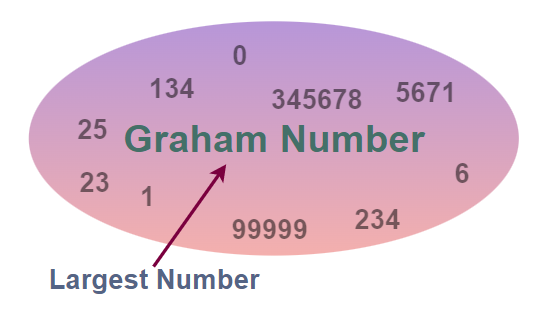GeeksforGeeks App
Open AppBrowser
Continue

# What is the largest number in the world?

We can not directly give the answer to this question of what is the largest number in the world as there are infinite numbers and the largest number can never be written because we can always add 1 to any large number. So the numbers tend to go to infinity. And infinity is not any number but a concept.

## What is the Largest Number in the World?

A Googolplex is considered to be the biggest number in the world. It is written as 10googol. The number 10googol can also be expressed in the exponential format that will equal. While writing the number googol in normal number writing format the probability of losing count is very high. Hence, it is more convenient to express it in the form of exponent and powers.

The number googolplex is so ridiculously large that it is unimaginable to be written down in number writing format. The number hence remains a concept as it is of no use in mathematics for basic calculations.

So now one might ask what about, Googolpex Plus One, and so on they are bigger than Googolpex, that is right but we define a large number with some specific property and adding one to any large number is not accepted.

So what about Infinity? Now infinity is not a number but is rather a concept. One might argue can’t define more numbers greater than Googolplex in any way. The answer to this is yes we can, and Graham’s Number is one such number. It is exponentially bigger than Googolpex.

## How Big is Graham’s Number?

Graham’s Number is ridiculously big it is so big that we can not even imagine that number and that is not enough number in the observable number in the universe to even write that number.It was named after famous mathematician Ronald Graham, who gave the concept of this number. Graham’s number is so large that we cannot write it in standard notation, but it can be expressed using mathematical symbols and notation.

## FAQs

### Q1: Which number in the world holds a Guinness World Record?

Graham’s number holds the Guinness World Record for the biggest specific integer used in a published mathematical proof.

### Q2: Who attempted to write down the number Googolplex?

A mathematician Wolfgang H Nitsche attempted to write down the number googolplex and started releasing editions of a book of it.

### Q3: What is a Sextillion?

A sextillion is a number counting one million million million million million million, in the exponential form it is written as  1036 for the long-scale counting. The number is symbolically represented by SX.

### Q4: How many Billions equal One Quadrillion?

1000000 billion is equal to one quadrillion.